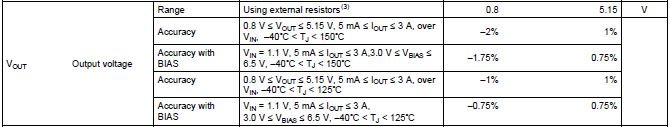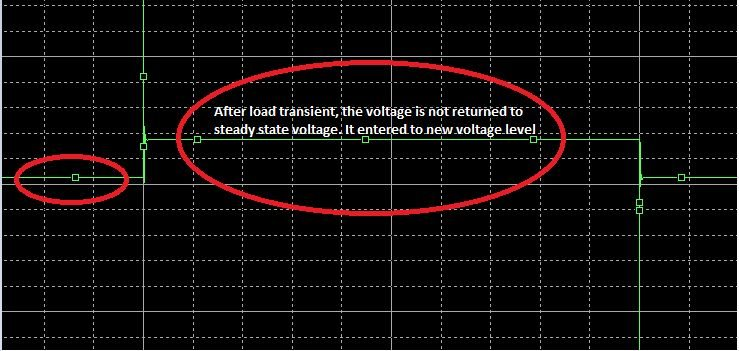If you have a related question, please click the "Ask a related question" button in the top right corner. The newly created question will be automatically linked to this question.

# TPS7A53-Q1: Queries in the datasheet

Part Number: TPS7A53-Q1

Hi,

I have following queries on TPS7A53-Q1

1)In the datasheet pg no:6, accuracy of output voltage is provided.

a)What is accuracy with Bias? Is it when bias voltage is used

b)In the accuracy with Bias for 125C Junction, -0.75% and 75% is provided. Whether this -0.75% and 0.75% is the accuracy of internal reference voltage(0.8V)? If not, what is the variation of 0.8V?2)When the input voltage is above 3.3V, is it ok to use bias voltage?

3)What is the maximum limit of soft -start voltage? When simulated using the Pspice model, it is found that the voltage on SS pin capacitor keeps on increasing.

4)Whether using 12.7kohm resistor instead of 12.1kohm resistor will cause any problem?

Thanks

Viswa

• Hi Viswa,

See my responses to your questions below, I've put my responses in green to make them easy to find

1)In the datasheet pg no:6, accuracy of output voltage is provided.

a)What is accuracy with Bias? Is it when bias voltage is used

Yes, you are correct any time you seethe notation "with Bias" that means that a bias voltage was provided.

b)In the accuracy with Bias for 125C Junction, -0.75% and 75% is provided. Whether this -0.75% and 0.75% is the accuracy of internal reference voltage(0.8V)? If not, what is the variation of 0.8V?

The +-0.75% accuracy shows how much error there will be at the output of the LDO, so these specs remove the any inaccuracy due to external resistors. The reason we give this value and not the accuracy of the internal voltage reference (0.8V) is because the internal reference has a much tighter accuracy but ultimately a user needs to know how the output voltage will behave since there are other sources of error beyond just the reference voltage.2)When the input voltage is above 3.3V, is it ok to use bias voltage?

Yes you can connect a bias voltage when Vin>3.3V, many customers do this and it will not alter the performance of the LDO.

3)What is the maximum limit of soft -start voltage? When simulated using the Pspice model, it is found that the voltage on SS pin capacitor keeps on increasing.

Yes, the model is behavioral and doesn't always mimic every aspect of the actual LDO. The voltage on the SS pin is the reference voltage so the maximum you will see on this pin is 0.8V plus the max accuracy spec which aligns with your application.  So in the worst case you should not see more than ~810mV.

4)Whether using 12.7kohm resistor instead of 12.1kohm resistor will cause any problem?

No this will not cause any problems. 12.1k is recommended to have a similar impedance as the internal node however small changes in the external resistor will not noticeably affect the PSRR and Noise performance. Also note that the internal impedance will vary due to normal process variation which is likely to see worst case value shifts of+-20%.

• Hi Kyle,

One query on the second point and the third point

a) I can understand that the +/-0.75% is the accuracy at the output of the LDO (without including external factors). That means, the voltage at the FB pin can be 0.794V (calculated by: 0.8V*(1-0.75%)) and 0.806V (calculated by: 0.8V*(1+0.75%)) . Is this correct?

b)810mV. Is this approximately calculated with Accuracy specification(i.e below table)? If my case is, +/0.75% , then maximum soft-start voltage is 0.806V.c)Whether we can use the model available in the TI website to do load and line transient simulation? The load transient simulation was performed. When the current value is increased, the output voltage is  increased after the overshoot. When the current value is decreased, the output voltage is decreased after the undershoot. The LDO is not regulating the voltage at constant value.Thanks

Viswa

• Hey Viswa,

a) I can understand that the +/-0.75% is the accuracy at the output of the LDO (without including external factors). That means, the voltage at the FB pin can be 0.794V (calculated by: 0.8V*(1-0.75%)) and 0.806V (calculated by: 0.8V*(1+0.75%)) . Is this correct?

Yes you are correct.

b)810mV. Is this approximately calculated with Accuracy specification(i.e below table)? If my case is, +/0.75% , then maximum soft-start voltage is 0.806V.

Correctc)Whether we can use the model available in the TI website to do load and line transient simulation? The load transient simulation was performed. When the current value is increased, the output voltage is  increased after the overshoot. When the current value is decreased, the output voltage is decreased after the undershoot. The LDO is not regulating the voltage at constant value.I'm unsure what the scale is on the image you showed of the model's line transient, however their is typically a slight change in the DC output voltage for different loads. In fact most EC Tables also provide information about load regulation, which is how much the output is expected to change for a given change in load, to help customers understand how the output voltage will change vs load (see screen shot from TPS7A53-Q1 datasheet). Note that for the TPS7A53-Q1 this change in output voltage is included in the overall accuracy spec since the accuracy spec applies to the entire range of loads (not all LDOs are specified this way).

The TPS7A53-Q1 datasheet also shows the behavior you described in the load transient plots in the char graph section of the datasheet, below is a screenshot of the relevant graph.